# AVL的特性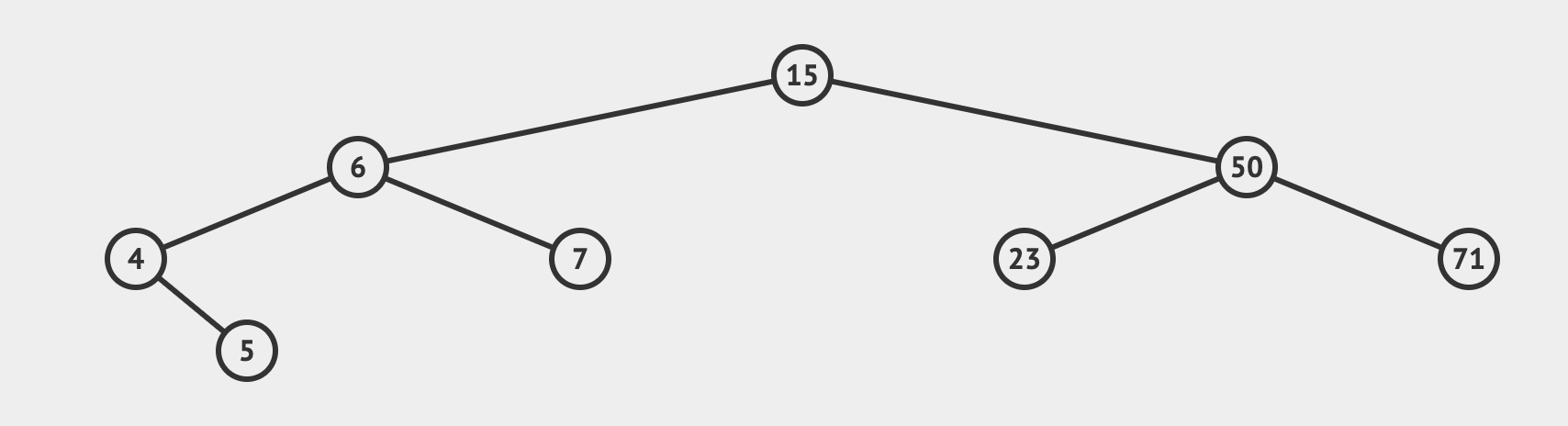# AVL的构建

``````public class AVLTree {

//根节点
Node root;

class Node {
int data; //节点的数据
int height; //节点的高度
Node left;
Node right;

public Node(int data) {
this.data = data;
left = right = null;
}
}
``````

``````//获取给定节点的高度
int height(Node node) {
if (node == null)
return 0;
return node.height;
}
``````

``````//获取平衡因子
int getBalance(Node node) {
if (node == null)
return 0;
return height(node.left) - height(node.right);
}
``````

# AVL的搜索

AVL的搜索和二叉搜索树的搜索方式是一致的。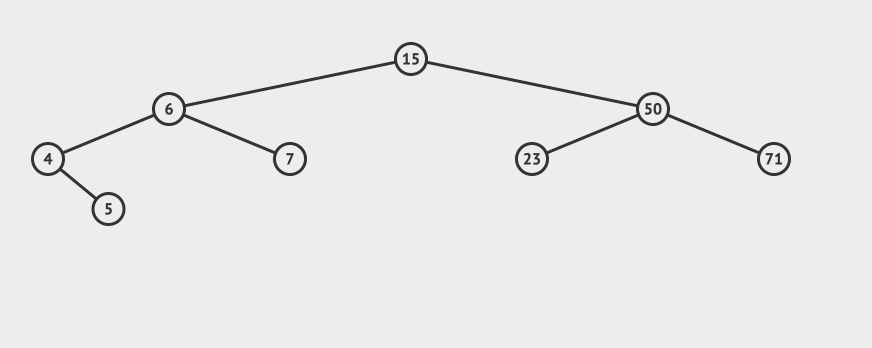1. 从根节点15出发，比较根节点和搜索值的大小
2. 如果搜索值小于节点值，那么递归搜索左侧树
3. 如果搜索值大于节点值，那么递归搜索右侧树
4. 如果节点匹配，则直接返回即可。

``````//搜索方法，默认从根节点搜索
public Node search(int data){
return search(root,data);
}

//递归搜索节点
private Node search(Node node, int data)
{
// 如果节点匹配，则返回节点
if (node==null || node.data==data)
return node;

// 节点数据大于要搜索的数据，则继续搜索左边节点
if (node.data > data)
return search(node.left, data);

// 如果节点数据小于要搜素的数据，则继续搜索右边节点
return search(node.right, data);
}
``````

# AVL的插入

AVL的插入和BST的插入是一样的，不过插入之后有可能会导致树不再平衡，所以我们需要做一个再平衡的步骤。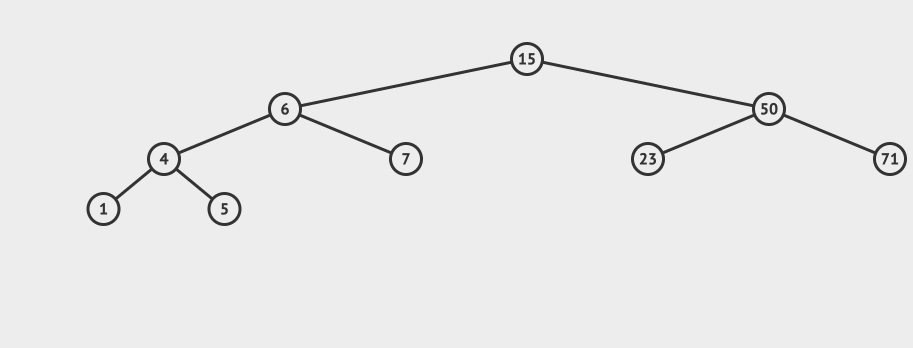1. 从根节点出发，比较节点数据和要插入的数据
2. 如果要插入的数据小于节点数据，则递归左子树插入
3. 如果要插入的数据大于节点数据，则递归右子树插入
4. 如果根节点为空，则插入当前数据作为根节点

1. 从插入的节点向上找出第一个未平衡的节点，这个节点我们记为z
2. 对z为根节点的子树进行旋转，得到一个平衡树。

• left-left：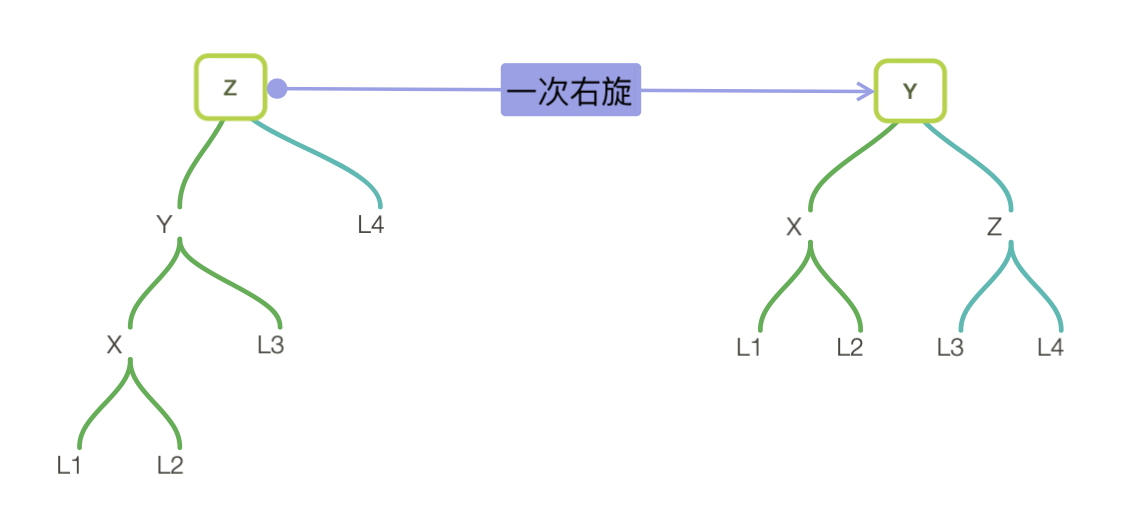1. 找到z节点的左节点y
2. 将y作为旋转后的根节点
3. z作为y的右节点
4. y的右节点作为z的左节点
5. 更新z的高度

``````Node rightRotate(Node node) {
Node x = node.left;
Node y = x.right;

// 右旋
x.right = node;
node.left = y;

// 更新node和x的高度
node.height = max(height(node.left), height(node.right)) + 1;
x.height = max(height(x.left), height(x.right)) + 1;

// 返回新的x节点
return x;
}
``````
• right-right: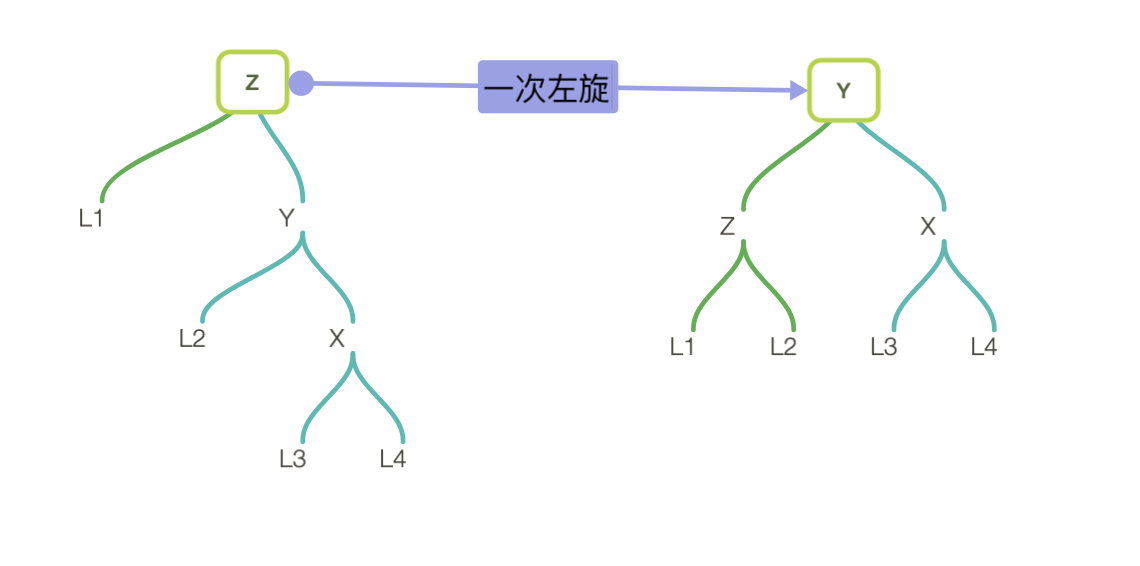1. 找到z节点的右节点y
2. 将y作为旋转后的根节点
3. z作为y的左节点
4. y的左节点作为z的右节点
5. 更新z的高度

``````//左旋
Node leftRotate(Node node) {
Node x = node.right;
Node y = x.left;

//左旋操作
x.left = node;
node.right = y;

// 更新node和x的高度
node.height = max(height(node.left), height(node.right)) + 1;
x.height = max(height(x.left), height(x.right)) + 1;

// 返回新的x节点
return x;
}
``````
• left-right：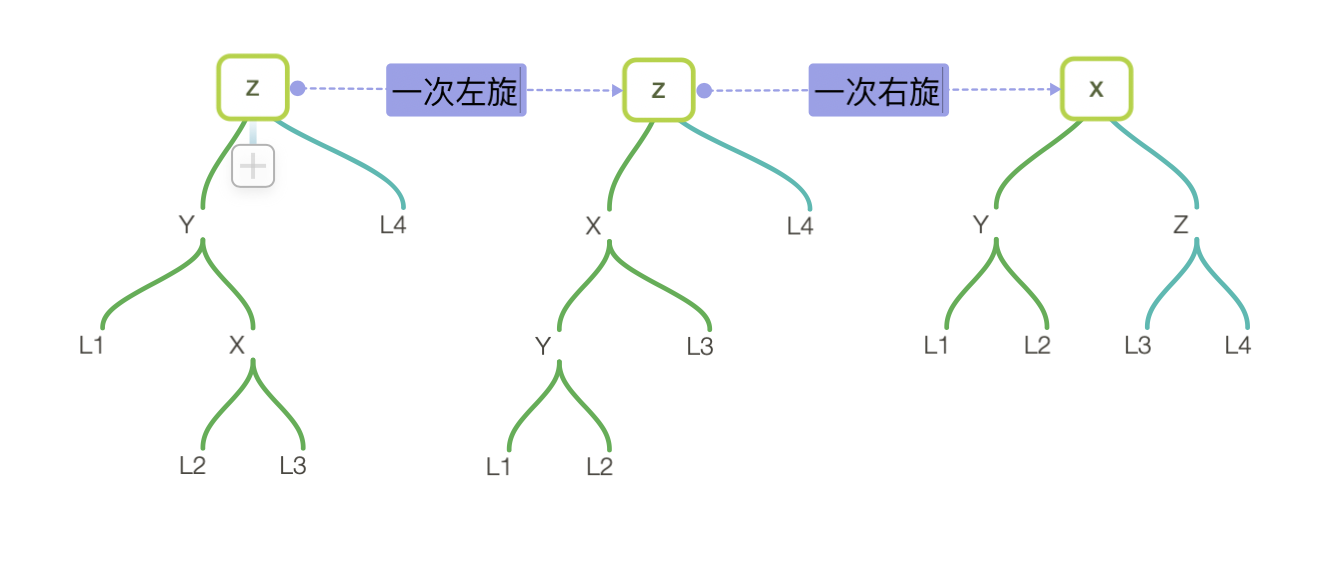• right-left：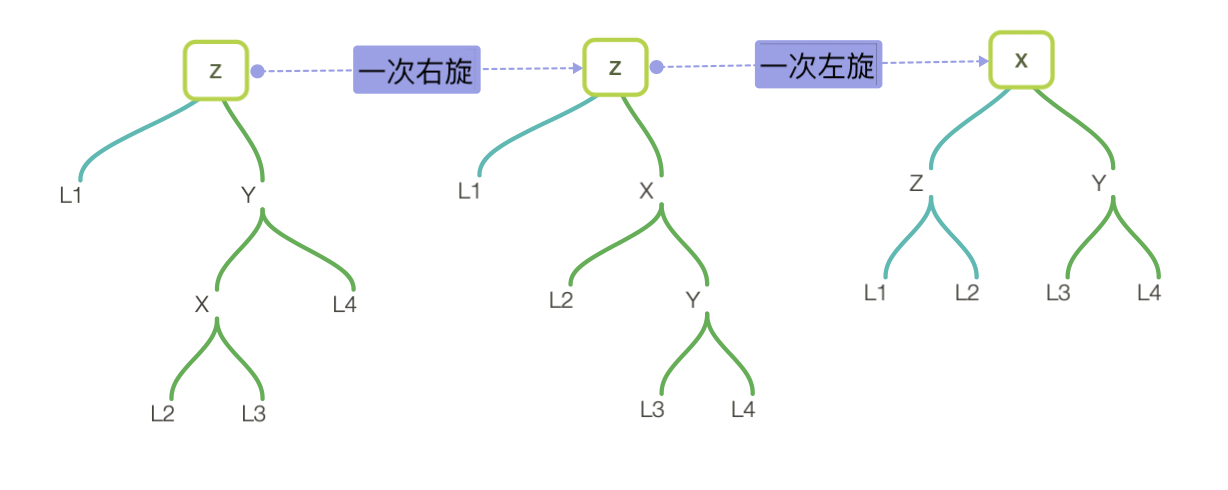1. 如果balance>1,那么我们在Left Left或者left Right的情况，这时候我们需要比较新插入的data和node.left.data的大小

如果data < node.left.data，表示是left left的情况，只需要一次右旋即可

如果data > node.left.data，表示是left right的情况，则需要将node.left进行一次左旋，然后将node进行一次右旋

2. 如果balance<-1,那么我们在Right Right或者Right Left的情况，这时候我们需要比较新插入的data和node.right.data的大小
如果data > node.right.data，表示是Right Right的情况，只需要一次左旋即可

如果data < node.left.data，表示是Right left的情况，则需要将node.right进行一次右旋，然后将node进行一次左旋

``````//插入新节点，从root开始
public void insert(int data){
root=insert(root, data);
}

//遍历插入新节点
Node insert(Node node, int data) {

//先按照普通的BST方法插入节点
if (node == null)
return (new Node(data));

if (data < node.data)
node.left = insert(node.left, data);
else if (data > node.data)
node.right = insert(node.right, data);
else
return node;

//更新节点的高度
node.height = max(height(node.left), height(node.right)) + 1;

//判断节点是否平衡
int balance = getBalance(node);

//节点不平衡有四种情况
//1.如果balance>1,那么我们在Left Left或者left Right的情况，这时候我们需要比较新插入的data和node.left.data的大小
//如果data < node.left.data，表示是left left的情况，只需要一次右旋即可
//如果data > node.left.data，表示是left right的情况，则需要将node.left进行一次左旋，然后将node进行一次右旋
//2.如果balance<-1,那么我们在Right Right或者Right Left的情况，这时候我们需要比较新插入的data和node.right.data的大小
//如果data > node.right.data，表示是Right Right的情况，只需要一次左旋即可
//如果data < node.left.data，表示是Right left的情况，则需要将node.right进行一次右旋，然后将node进行一次左旋

//left left
if (balance > 1 && data < node.left.data)
return rightRotate(node);

// Right Right
if (balance < -1 && data > node.right.data)
return leftRotate(node);

// Left Right
if (balance > 1 && data > node.left.data) {
node.left = leftRotate(node.left);
return rightRotate(node);
}

// Right Left
if (balance < -1 && data < node.right.data) {
node.right = rightRotate(node.right);
return leftRotate(node);
}

//返回插入后的节点
return node;
}
``````

# AVL的删除

AVL的删除和插入类似。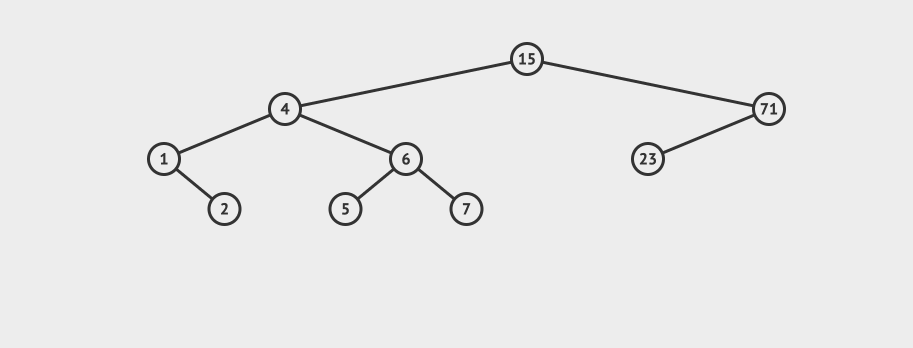1. 如果balance>1,那么我们在Left Left或者left Right的情况，这时候我们需要比较左节点的平衡因子

如果左节点的平衡因子>=0，表示是left left的情况，只需要一次右旋即可

如果左节点的平衡因<0，表示是left right的情况，则需要将node.left进行一次左旋，然后将node进行一次右旋

2. 如果balance<-1,那么我们在Right Right或者Right Left的情况，这时候我们需要比较右节点的平衡因子

如果右节点的平衡因子<=0，表示是Right Right的情况，只需要一次左旋即可

如果右节点的平衡因子>0，表示是Right left的情况，则需要将node.right进行一次右旋，然后将node进行一次左旋

``````Node delete(Node node, int data)
{
//Step 1. 普通BST节点删除
// 如果节点为空，直接返回
if (node == null)
return node;

// 如果值小于当前节点，那么继续左节点删除
if (data < node.data)
node.left = delete(node.left, data);

//如果值大于当前节点，那么继续右节点删除
else if (data > node.data)
node.right = delete(node.right, data);

//如果值相同，那么就是要删除的节点
else
{
// 如果是单边节点的情况
if ((node.left == null) || (node.right == null))
{
Node temp = null;
if (temp == node.left)
temp = node.right;
else
temp = node.left;

//没有子节点的情况
if (temp == null)
{
node = null;
}
else // 单边节点的情况
node = temp;
}
else
{  //非单边节点的情况
//拿到右侧节点的最小值
Node temp = minValueNode(node.right);
//将最小值作为当前的节点值
node.data = temp.data;
// 将该值从右侧节点删除
node.right = delete(node.right, temp.data);
}
}

// 如果节点为空，直接返回
if (node == null)
return node;

// step 2: 更新当前节点的高度
node.height = max(height(node.left), height(node.right)) + 1;

// step 3: 获取当前节点的平衡因子
int balance = getBalance(node);

// 如果节点不再平衡，那么有4种情况
//1.如果balance>1,那么我们在Left Left或者left Right的情况，这时候我们需要比较左节点的平衡因子
//如果左节点的平衡因子>=0，表示是left left的情况，只需要一次右旋即可
//如果左节点的平衡因<0，表示是left right的情况，则需要将node.left进行一次左旋，然后将node进行一次右旋
//2.如果balance<-1,那么我们在Right Right或者Right Left的情况，这时候我们需要比较右节点的平衡因子
//如果右节点的平衡因子<=0，表示是Right Right的情况，只需要一次左旋即可
//如果右节点的平衡因子>0，表示是Right left的情况，则需要将node.right进行一次右旋，然后将node进行一次左旋
// Left Left Case
if (balance > 1 && getBalance(node.left) >= 0)
return rightRotate(node);

// Left Right Case
if (balance > 1 && getBalance(node.left) < 0)
{
node.left = leftRotate(node.left);
return rightRotate(node);
}

// Right Right Case
if (balance < -1 && getBalance(node.right) <= 0)
return leftRotate(node);

// Right Left Case
if (balance < -1 && getBalance(node.right) > 0)
{
node.right = rightRotate(node.right);
return leftRotate(node);
}
return node;
}
``````

learn-algorithm